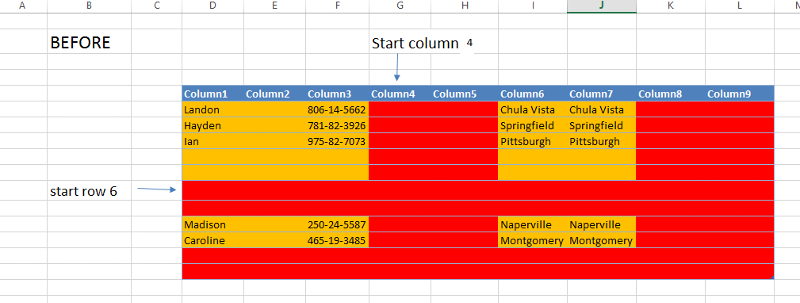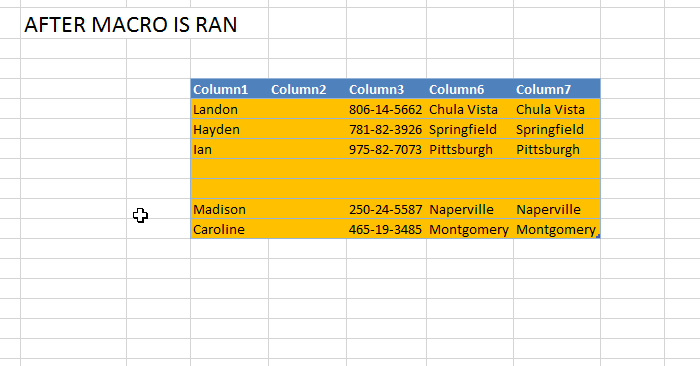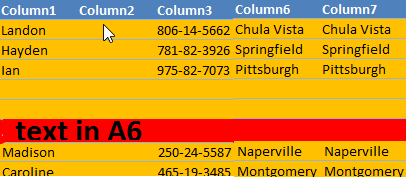help with VBA on Excel TablesFlora Edwards used Ask the Experts™
on
I have been trying myself to find a solution based on couple of codes i have found on net.

basically what i need is

i have more than 50 tables in many worksheets on my workbook.

each of the tables have empty rows and empty columns.

some empty columns or rows are in between and some are at the end of the data.

now what i need is that for all of these tables, i want the delete operation to start from #x number of rows or columns and this #x numbe r of row and columns should be given by inputbox by user.

so in more simple way.

when i run the macro.
it should ask me via inputbox to enter the number of starting ROW on tables based on where the delete of empty cells should start
then it asks me via inputbox to enter the number of starting COLUMN on tables based on where the delete of empty cells should start.
after that it loops through all of the tables in active workbook and deletes the empty rows and columns.
for example if on first inputbox i put 4 and next inputbox i put 3 then macro should start checking the empty cells from row 4 and from column 3

thanks.

for your convenience, i have uploaded a dummy file.

also the below codes from net, which i could not modify to get it worked.

example
Public Sub DeleteLastRow()
Const DELETE_ONLY_IF_BLANK = True               '  This flag if set to FALSE will ensure a non-blank row is also deleted
Const CHECK_ONE_COLUMN_LESS = True              '  This flag if set to TRUE will delete only if all columns are blank

Dim oLst As ListObject
Dim Number_of_rows As Long
Dim Number_of_columns As Integer

Application.ScreenUpdating = False

For Each oLst In ActiveSheet.ListObjects
With oLst
If .Name = "Table3" Then               '  Change the table name to suit
Number_of_columns = .ListColumns.Count
Number_of_rows = .ListRows.Count
If Number_of_rows > 1 Then
If DELETE_ONLY_IF_BLANK Then
If Application.WorksheetFunction.CountBlank(.ListRows(Number_of_rows).Range.Offset(, 1).Resize(, Number_of_columns + 1 * CHECK_ONE_COLUMN_LESS)) = Number_of_columns + 1 * CHECK_ONE_COLUMN_LESS Then
.ListRows(Number_of_rows).Delete
End If
Else
.ListRows(Number_of_rows).Delete
End If
End If
.ListRows(1).Range.ClearContents
End If
End With
Next
Application.ScreenUpdating = True
End Sub
TB.xlsm
Comment
Watch Question

Do more withEXPERT OFFICE® is a registered trademark of EXPERTS EXCHANGE®
When you say deletes empty rows and columns, what exactly do you mean.

EG in your example if row 5 was empty should row 6 be moved up to row 5 etc, what happens to data in the old row 5 prior to column 3. The same for columns, if column 7 was empty do all columns to the right get moved across 1 and what happens to all cells in column 7 above row 4?
Medicine

Commented:
thank you ChloesDad for helping me on this.

i have made two screenshot for an example senario.

Now lets say that on the macro input-box  for row i put 6 meaning to start from 6th row as shown in picture below and on the next input-box for column, i put 4 meaning to start from 4th column.AFTER MACRO is ran then the result should look like the screenshot below.as you can see in the screenshots above.  any empty row or column after the starting row and column has been deleted and data other data moved up and left.
You haven't answered about data in column 3 row 6, is that just deleted as well even if it das data in it? Your example shows it empty anyway, but what if it does have data in it?
Medicine

Commented:
if it has data in it, then it should not delete. it only should delete those which are empty.

from the two screenshots above you can see that those rows and columns highlighted in red are deleted and they were all blanks. none of them had data
OK What happens in this example
MyExample.png
Medicine

Commented:
in that case that row with text remains. becuase that row was not empty and it had a text on it and could not be called empty row.I think I misunderstood the question.

You want to remove empty rows after row x and empty columns after column y. Not just remove rows after row x that are empty after column y, and columns after y that are empty after row x. my bad
Medicine

Commented:
Yes
Medicine

Commented:
I've requested that this question be deleted for the following reason:

Medicine

Commented:
re-opened as Rgonzo will try to help me on this.
Top Expert 2016

Commented:
The problem in you example there is multiple tables in the sheet then it won't work the problem comes when different Tables have a common row or a common column
Medicine

Commented:
thanks Rgonzo

lets forget about multiple tables in single worksheets,

would it be possible if i have one table in each sheet?
Top Expert 2016
Commented:
then try

Sub macro()

InputCol = Application.InputBox("Enter the first column to be deleted", "Enter Col Number", , , , , , 1)
FirstCol = Abs(Int(InputCol))
If FirstCol = 0 Then Exit Sub
InputRow = Application.InputBox("Enter the first row to be deleted", "Enter Row Number", , , , , , 1)
FirstRow = Abs(Int(InputRow))
If FirstRow = 0 Then Exit Sub

For Each sh In ActiveWorkbook.Sheets
For Each tbl In sh.ListObjects
For Idx = tbl.ListRows.Count To FirstRow Step -1
If WorksheetFunction.CountBlank(tbl.ListRows(Idx).Range) = tbl.ListRows(Idx).Range.Cells.Count Then
tbl.ListRows(Idx).Range.EntireRow.Delete
End If
Next
For Idx = tbl.ListColumns.Count To FirstCol Step -1
If WorksheetFunction.CountBlank(tbl.ListColumns(Idx).DataBodyRange) = tbl.ListColumns(Idx).DataBodyRange.Cells.Count Then
tbl.ListColumns(Idx).DataBodyRange.EntireColumn.Delete
End If
Next
Next
Next
End Sub
Medicine

Commented:
remarkable.  thanks alot

Do more withSubmit tech questions to Ask the Experts™ at any time to receive solutions, advice, and new ideas from leading industry professionals.